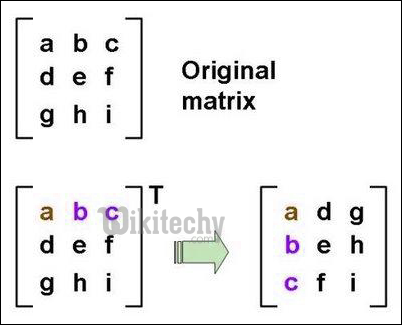# python tutorial - Transpose Matrix | Transpose a matrix in Single line in Python - learn python - python programmingLearn Python - Python tutorial - python matrix - Python examples - Python programs

Transpose of a matrix is a task we all can perform very easily in python (Using a nested loop). But there are some interesting ways to do the same in a single line.
In Python, we can implement a matrix as nested list (list inside a list). Each element is treated as a row of the matrix. For example m = [[1, 2], [4, 5], [3, 6]] represents a matrix of 3 rows and 2 columns.
First element of the list – m and element in first row, first column – m.1. Using Nested List Comprehension: Nested list comprehension are used to iterate through each element in the matrix.In the given example ,we iterate through each element of matrix (m) in column major manner and assign the result to rez matrix which is the transpose of m.

python - Sample - python code :

python tutorial - Output :

1. Using zip: Zip returns an iterator of tuples, where the i-th tuple contains the i-th element from each of the argument sequences or iterables. In this example we unzip our array using * and then zip it to get the transpose.

python - Sample - python code :

python tutorial - Output :

Note :- If you want your result in the form [[1,4,7,10][2,5,8,11][3,6,9,12]] , you can use t_matrix=map(list, zip(*matrix)).

1. Using numpy: NumPy is a general-purpose array-processing package designed to efficiently manipulate large multi-dimensional arrays. The transpose method returns a transposed view of the passed multi-dimensional matrix.

python - Sample - python code :

Wikitechy tutorial site provides you all the learn python , python code , python script , python windows , python mysql , django python , python django , python compiler , jython , python interpreter , what is python , python join , python input , python modules , python editor , python examples , python pdf , python documentation